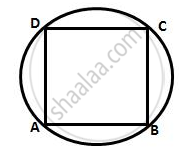Share

Prove That: the Rhombus, Inscribed in a Circle, is a Square. - ICSE Class 10 - Mathematics

ConceptArc and Chord Properties - Angle in a Semi-circle is a Right Angle

Question

Prove that:
the rhombus, inscribed in a circle, is a square.

SolutionLet ABCD be a rhombus, inscribed in a circle
Now, ∠BAD = ∠BCD
(Opposite angles of a parallelogram are equal)
And ∠BAD = ∠BCD =180°
(pair of opposite angles in a cyclic quadrilateral are supplementary)
∴ ∠BAD = ∠ BCD (180°)/2=90°
∥y, the other two angles are 90° and all the sides
are equal.
∴ ABCD is a square.

Is there an error in this question or solution?

Video TutorialsVIEW ALL 

Solution Prove That: the Rhombus, Inscribed in a Circle, is a Square. Concept: Arc and Chord Properties - Angle in a Semi-circle is a Right Angle.
S